Salts of A (atomic weight 7), B (atomic weight = 27) and C (atomic weight 48) were electrolysed under identical conditions using same quantity of electricity. It was found that when 2.1g of A was deposited, weights of B and C deposited were 2.7g and 7.2g. The valencies of A, B and C are respectively.

Anonymous User Chemistry 07 Apr, 2020 73 views

A charged ball B hangs from a silk thread S, which makes an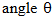with a large charged conducting sheet P, as shown in the figure. The surface charge density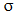of the sheet is proportional to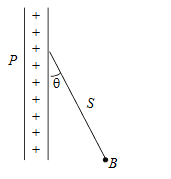Anonymous User Physics Electrostatic 07 Apr, 2020 76 views

A charged particle q is shot towards another charged particle Q which is fixed, with a speed v. It approaches Q upto a closest distance r and then returns. If q were given a speed 2v, what will b the closest distances of approach?

Anonymous User Physics Electrostatic 07 Apr, 2020 59 views

Two spherical conductors B and C having equal radii and carrying equal charges in them repel each other with a force F when kept apart at some distance. A third spherical conductor having the same radius as that of B but uncharged is brought in contact with B, then brought in contact with and finally removed away from both. The new force of repulsion between B and C is

Anonymous User Physics Electrostatic 07 Apr, 2020 81 views

Three charges –q1, +q2 and –q3 are placed as shown in the figure. The x-­component of the force on –q1 is proportional to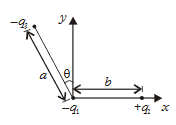Anonymous User Physics Electrostatic 07 Apr, 2020 63 views

A thin spherical conducting shell of radius R has a charge q. Another charge Q is placed at the centre of the shell. The electrostatic potential at a point P at a distance R/2 from the centre of the shell is

Anonymous User Physics Electrostatic 07 Apr, 2020 63 views

If Ihe electric flux entering and leaving an enclosed surface respectively is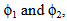the electric charge inside the surface will be

Anonymous User Physics Electrostatic 07 Apr, 2020 60 views

A charged particle q is placed at the centre O of cube of length L (ABCDEFGH). Another same charge q is placed at a distance from O. Then the electric flux through ABCD is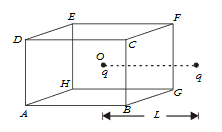Anonymous User Physics Electrostatic 07 Apr, 2020 57 views

The equations 5x2 + 12x + 13 = 0 and ax2 + bx + c = 0 (a,b,c  belongs R) have a common root, where a, b, c are the sides of the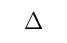ABC. Then find C .

Anonymous User Maths Quadratic Equation 06 Apr, 2020 86 views

## The value of the expression x2 + 2bx + c will be positive for all real x ifThe value of the expression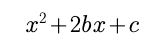will be positive for all real x if

Anonymous User Maths Quadratic Equation 06 Apr, 2020 404 views

What is spermatogenesis? Which major events take place

during the following stages of formation of spermatids?

(a) Multiplication phase

(b) Growth phase

(c) Maturation phase

Anonymous User Biology Human Reproduction 05 Apr, 2020 67 views